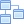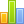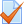# Mathematics 101

Mathematics is the study of topics such as quantity (numbers), structure, space, and change.

## Content

•Logic

The concept of logical form is central to logic. The validity of an argument is determined by its logical form, not by its content. Traditional Aristotelian syllogistic logic and modern symbolic logic are examples of formal logic.

• Informal logic is the study of natural language arguments. The study of fallacies is an important branch of informal logic. Since much informal argument is not strictly speaking deductive, on some conceptions of logic, informal logic is not logic at all. See 'Rival conceptions', below.
• Formal logic is the study of inference with purely formal content. An inference possesses a purely formal content if it can be expressed as a particular application of a wholly abstract rule, that is, a rule that is not about any particular thing or property. The works of Aristotle contain the earliest known formal study of logic. Modern formal logic follows and expands on Aristotle. In many definitions of logic, logical inference and inference with purely formal content are the same. This does not render the notion of informal logic vacuous, because no formal logic captures all of the nuances of natural language.
• Symbolic logic is the study of symbolic abstractions that capture the formal features of logical inference. Symbolic logic is often divided into two main branches: propositional logic and predicate logic.
• Mathematical logic is an extension of symbolic logic into other areas, in particular to the study of model theoryproof theoryset theory, and recursion theory.

However, agreement on what logic is has remained elusive, and although the field of universal logic has studied the common structure of logics, in 2007 Mossakowski et al. commented that "it is embarrassing that there is no widely acceptable formal definition of 'a logic'"

•How strong is your foundational knowledge?

Applied mathematics is the application of mathematical methods by different fields such as scienceengineeringbusinesscomputer science, and industry. Thus, applied mathematics is a combination of mathematical scienceand specialized knowledge. The term "applied mathematics" also describes the professional specialty in which mathematicians work on practical problems by formulating and studying mathematical models. In the past, practical applications have motivated the development of mathematical theories, which then became the subject of study in pure mathematics where abstract concepts are studied for their own sake. The activity of applied mathematics is thus intimately connected with research in pure mathematics.

Elementary algebra differs from arithmetic in the use of abstractions, such as using letters to stand for numbers that are either unknown or allowed to take on many values. For example, in $x+2=5$the letter $x$is unknown, but the law of inverses can be used to discover its value: $x=3$. In E = mc2, the letters $E$and $m$are variables, and the letter $c$is a constant, the speed of light in a vacuum. Algebra gives methods for writing formulas and solving equations that are much clearer and easier than the older method of writing everything out in words.

The word algebra is also used in certain specialized ways. A special kind of mathematical object in abstract algebra is called an "algebra", and the word is used, for example, in the phrases linear algebra and algebraic topology.

Calculus is the mathematical study of continuous change, in the same way that geometry is the study of shape and algebra is the study of generalizations of arithmetic operations. It has two major branches, differential calculus (concerning rates of change and slopes of curves), and integral calculus (concerning accumulation of quantities and the areas under and between curves). These two branches are related to each other by the fundamental theorem of calculus. Both branches make use of the fundamental notions of convergence of infinite sequences and infinite series to a well-defined limit. Generally, modern calculus is considered to have been developed in the 17th century by Isaac Newton and Gottfried Wilhelm Leibniz. Today, calculus has widespread uses in scienceengineering, and economics

•Final Exam

0
0 Reviews